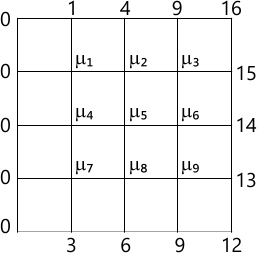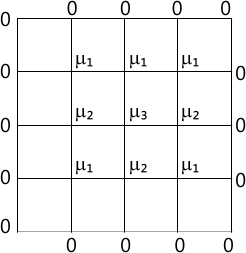MORE IN Engineering Mathematics 3
VTU Electronics and Communication Engineering (Semester 3)
Engineering Mathematics 3
December 2014
Total marks: --
Total time: --
INSTRUCTIONS
(1) Assume appropriate data and state your reasons
(2) Marks are given to the right of every question
(3) Draw neat diagrams wherever necessary

1(a) Expand $F(X)=\sqrt{1-cosx,},0< X < 2\pi$ in a Fourier series.Hence evaluate $\frac{1}{1.3}+\frac{1}{3.5}+\frac{1}{5.7}+...$
7 M
1(b) Find the half - range sine series of [f(x)=e^{x}=e ] in (0,1).
6 M
1(c) In a machine the displacement y of a given for a certain angle x as follows:
 x 0 30 60 90 120 150 180 210 240 270 300 330 y 7.9 8 7.2 5.6 3.6 1.7 0.5 0.2 0.9 2.5 4.7 6.8

Find the constant term and the two harmonics in Fourier series expansion of y.
7 M

2(a) Find Fourier transform$e^{-}|^{x}|$ and hence evaluate$\int_{0}^{\oe }\frac{cosxt}{1+t^{2}}dt.$
7 M
2(b) Find Fourier sine transform of$F(x)=\left\{\begin{matrix} x, &0< x\leq 1 \\ 2-x, &1\leq x< 2. \\0, &x> 2. \end{matrix}\right.$
6 M
2(c) Solve the integral equation$\int_{0}^{\oe }f(x)\lambda xdx=e^{-\lambda }.$
7 M

3(a) Find various possible solution of one-dimensional heat equation by separable variable method.
10 M
3(b) A rectangular plate with insulated surface is 10cm wide and so long compared to its width that it may be considered infinite in length without introducing an appreciable error.If the temperature of the short edge$y^{-0}$ is given by
$u^{-}20_{x}0\leq X\leq 5\\-20(10-X),5\leq X\leq 10$
and the two long edges X=0$^{0}$,X=10 as well as the other short edge are kept at 0C,find the temperature u(x,y)
10 M

4(a) Fit a curve of the form $y^{-}ae^{bx}$ to the data:
 x 1 5 7 9 12 y 10 15 12 15 21
7 M
4(b) use graphical method to solve the following LPP:
minimize$Z^{-}20_{x1}+30_{x2}\\ Subject\ to\ 2x_{1}+2x_{2}\leq 20;\\ 3x_{1},x_{2}\geq 0.$
6 M
4(c) Solve the following LPP by using simplex method.
maximize$Z-3_{x1}-2_{x2}+5_{x3}\\Subject\ to \ x^{1} 1 \ 2x_{2}\ x_{3} \leq 430\\3x_{1}-2x_{3\ \leq }\ 460\\ x_{1}\ \geq \ x_{2}\geq \ 0.$
7 M

5(a) Useing the Gauss-Seidal iterative method to solve the system of linear equations.27x+6y+z=85;6x+15y-2z=72;x-y+54z=110.Carry out 3 iterations by taking the initial approximation to the solution as (2,3,2). Consider four decimal places at each stage for each variable.
7 M
5(b) Using the Newton-Raphson method,find the real root of the equation xsinx+cosx-0 near to x$\pi$,carry out four iterations (x in radians).
6 M
5(c) Find the largest Eigen value and the corresponding Eigen vector of the matrix
A=\begin{pmatrix} 4 &1 &-1 \\2 &3 &-1 \\-2 &1 &5 \end{pmatrix}\] by power method. Take $\begin{pmatrix} 1\\0 \\0 \end{pmatrix}$as the initial vector.Perform 5 iterations.
7 M

6(a) Find f(0.1) by using Newton's forward interpolation formula and f(4.99)by using newton's backward interpolation formula form the data:
 x 0 1 2 3 4 5 f(x) -8 0 20 58 120 212
7 M
6(b) find the interpolating polynomial f(x) by using Newton's divided difference interpolation formula form the data;
 x 0 1 2 3 4 5 f(x) 3 2 7 24 59 118
6 M
6(c) Evaluate $\int_{0}^{2}e^{x}$dx using Weddle's rule.Taking six equal sub intervals,compare the result with exact value.
7 M

7(a) Solve $\frac{\partial^2 }{\partial x^2}+\frac{\partial^2u }{\partial y^2}-0$ in the following square mesh.carry out two iterations.7 M
7(b) Solve the Poisson's equation$\bigtriangledown ^{2}u=8x^{2}y^{2}$ for the square mesh given below with u-0 on the boundary and mesh length,h-1.6 M
7(c) Evaluate the pivotal values of $\frac{\partial^2 }{\partial xt^2}=16\frac{\partial^2u }{\partial x^2}$taking h-1 upto t-1.25. The boundary conditions are u(0,t),u(5,t)=0,$\frac{\partial u}{\partial t}(x,0)=0,u(x,0)=x^{2}(5-x).$
7 M

8(a) Find the Z-transforms of i)$\left ( \frac{1}{2} \right )^{n}+\left ( \frac{1}{3} \right )^{n}$
$3^{n}cos\frac{\pi n}{4}$
7 M
8(b) State and prove initial value theorem in Z-transforms.
6 M
8(c) Solve the difference equation
u_{n+2}-2u_{n+1}+u_{n};u_{n}=2,u_{1}=1\].
7 M

More question papers from Engineering Mathematics 3